## Resolving power

Diffraction of light through a rectangular aperture is a rather straightforward extension of 1-dimensional diffraction from a slit, as shown in the diagrams below.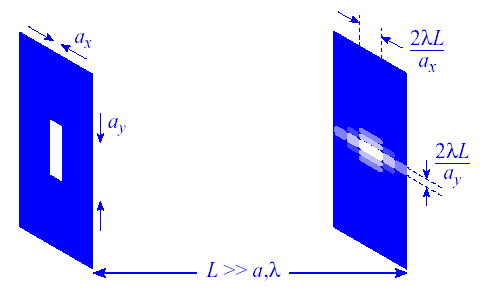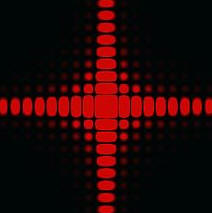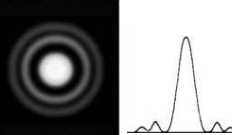A circular aperture is qualitatively similar, but an accurate quantitative treatment of the pattern requires more complicated mathematics.  The intensity pattern is called the "Airy Disk".  The main features are shown in the diagram below.  The first minimum occurs at an angle θ = 1.22 λ/D, where D is the diameter of the aperture.  On a screen a distance L >> D from the aperture the minimum is seen at a radial distance r' = 1.22 λL/D from the center of the pattern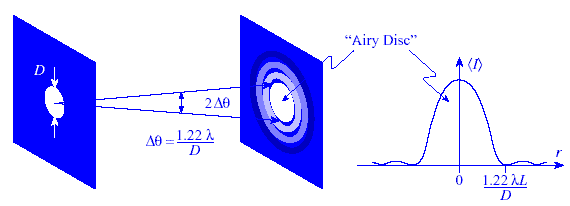Producing a laser beam is an attempt to confine the light in the directions transverse to the direction of propagation.  The light will spread out in the same way it does after passing through an aperture.

Assume that at z = 0 the diameter of a laser beam is restricted to a(0).  The angle through which the light spreads is approximately θ ≈ λ/a(0).  (For back-of-the-envelope calculations we often ignore the factor of 1.22.)  Because the laser beam diameter is typically much larger the wavelength of light, or a(0) >> λ, θ is quite small.  At a large distance z the diameter of the beam will have increased to  a(z) ≈ z*2θ.  Consider a HeNe laser, for which λ = 633 nm with a beam waist of ~ 0.6 mm.  Then θ ~ 10-3 rad = 1 millirad.  The beam must propagate ~ 3 m before the diameter increases by a factor of 10.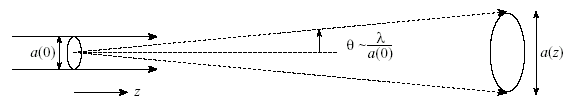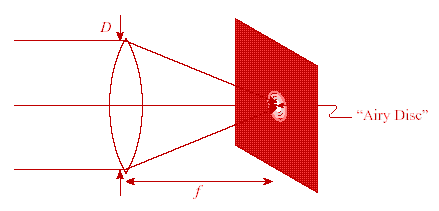In geometrical optics we assume that an ideal, aberration-free lens focuses parallel rays to a single point one focal length away from the lens.  But the lens itself acts like an aperture with diameter D for the incident light.  The light passing through the lens therefore spread out.  This yields a blurred spot at the focal point.  Light near the focal point exhibits an Airy Disc pattern.  The size of the Airy Disc is determined by the focal length f and diameter D of the lens.  The radius r of the Airy Disc at the focal point of a lens is given by r = 1.22 λf/D.

If all ray aberrations in an optical system can be eliminated, such that all of the rays leaving a given object point land inside of the Airy Disc associated with the corresponding image point, then we have a diffraction-limited optical system.  This is the absolute best we can do for an optical system that has lenses with finite diameters.

The resolving power of an optical instrument is its ability to separate the images of two objects, which are close together.  Some binary stars in the sky look like one single star when viewed with the naked eye, but the images of the two stars are clearly resolved when viewed with a telescope.

If you look at a far-away object, then the image of the object will form a diffraction pattern on your retina.  For two far-away objects, separated by a small angle θ, the diffraction patterns will overlap.  You are able to resolve the two objects as long as the central maxima of the two diffraction patterns do not overlap.  The two images are just resolved when one central maximum falls onto the first minimum of the other diffraction pattern.  This is known as the Rayleigh criterion.  If the two central maxima overlap the two objects look like one.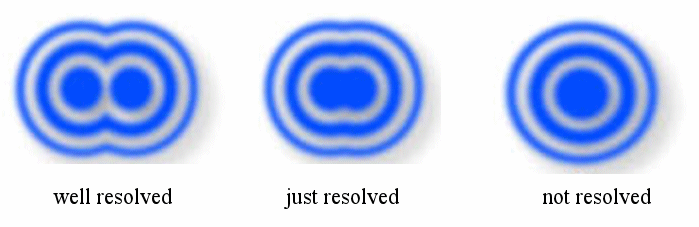The width of the central maximum in a diffraction pattern depends on the size of the aperture, (i.e. the size of the slit).  The aperture of your eye is your pupil.  A telescope has a much larger aperture, and therefore has a greater resolving power.  The minimum angular separation of two objects which can just be resolved is given by θmin = 1.22 λ/D, where D is the diameter of the aperture.  The factor of 1.22 applies to circular apertures like the pupil of your eye or the apertures in telescopes and cameras.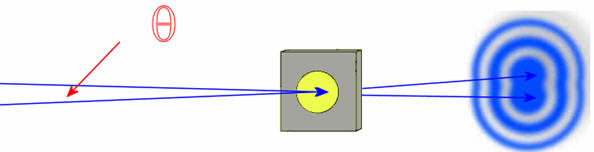When light passes through an aperture with diameter D, then diffraction limits the resolution to θ = 1.22λ/D.  If the angular separation of two sources is less than θ, they cannot be resolved.  The closer you are to two objects, the greater is the angular separation between them.  Up close, two objects are easily resolved.  As your distance from the objects increases, their images become less well resolved and eventually merge into one image.

#### Problem:

A spy satellite travels at a distance of 50 km above Earth's surface.  How large must the lens be so that it can resolve objects with a size of 2 mm and thus read a newspaper?  Assume the light has a wavelength of 400 nm.

Solution:

• Reasoning:
The minimum angular separation of two points which can just be resolved by an optical instrument is given by θmin = 1.22 λ/D, where D is the diameter of the aperture of the instrument.
• Details of the calculation:
Diffraction limits the resolution according to θ = 1.22 λ/D = y/L.  Here the height of the object to be resolved is y and the distance to the object is L.  Solving for D we find D = 12.2 m.

#### Module 9, Question 2

The limit to the eye's acuity is actually related to diffraction by the pupil.
Estimate the angle between two just-resolvable points of light for the pupil in bright daylight.  Make reasonable assumptions.
Given this angle, estimate the greatest possible distance you can resolve the two headlights of a car?

Discuss your estimates with your fellow students in the discussion forum!